# Integral of 1/x=ln(x), or

• DivergentSpectrum
In summary, In this conversation, the author found that ln(x) is always true, although it is not true when n = -1. Taking the integrand as x-1 at first results in a positive value, but as m approaches the negative side of zero, the value becomes negative. He found the average of both sides of the limit to be almost exactly ln(x), which he graphed and found to converge almost exactly to ln(x). Finally, he shared this information with other people, who mostly say that ln(x) is just ln(x), without mentioning the power property.f

#### DivergentSpectrum

so i was messing around and found that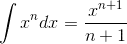is always true.
taking the integrand as x-1
at first we have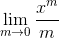but the limit is 2 sided(as m approaches the positive side of zero i get a positive value, while as m approaches the negative side of zero, i get a negative value.)

So i decided to find the average of both sides of the limit: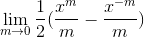i graphed this with m=a really small (positive) number and found that it converges almost exactly to ln(x)

anyway, i just thought it was pretty cool how the power property always holds so i figured id share it. most of the sites i see just say its ln(x), period.

Last edited:
so i was messing around and found thatis always true.
No, it's not true when n = -1.
DivergentSpectrum said:
taking the integrand as x-1
at first we havebut the limit is 2 sided(as m approaches the positive side of zero i get a positive value, while as m approaches the negative side of zero, i get a negative value.)
What does this have to do with the integral above? Furthermore, this limit does not exist.
DivergentSpectrum said:
So i decided to find the average of both sides of the limit:i graphed this with m=a really small (positive) number and found that it converges almost exactly to ln(x)

anyway, i just thought it was pretty cool how the power property always holds so i figured id share it. most of the sites i see just say its ln(x), period.

No, it's not true when n = -1.

in a way it is, i mean if its possible to find the integral with the standard power rule using limits, and that limit just happens to converge to ln(x) then its got to be true, right?
What does this have to do with the integral above? Furthermore, this limit does not exist.
its the limit form of x0/0
Ive heard people say that if the function is 2-sided the limit doesn't exist. But here the limit is clearly the average of both sides. I don't know if this is considered "standard" but obviously it has to mean something.'

its really pretty odd that wikipedia doesn't offeras an alternative definition for ln(x). it seems it would be pretty fundamental

Last edited:
so i was messing around and found thatis always true.

I'm sorry, but nothing you post will (or even can) support this claim. Whenever you write a fraction, say ##\frac{a}{b}##, the notation explicitly requires ##b\neq0##. This is the requirement of the notation. This means that from the right hand side of the equation, ##n\neq -1##, always. So the claim that "the equation is always true" is by default false.

Ive heard people say that if the function is 2-sided the limit doesn't exist.
Again by definition. A limit is said "to exist" if (a) it is independent of the path and (b) the value is an element of the co-domain.

its really pretty odd that wikipedia doesn't offeras an alternative definition for ln(x). it seems it would be pretty fundamental

Second, I certainly don't consider that fundamental.

note that i had to use rather large values of m (for m=.1 the difference is almost imperceptable to the eye)
so id have to say that not only does it converge, but it converges quite nicely as well.
and i derived it by evaluating a limit that (supposedly) doesn't exist.

#### Attachments

DivergentSpectrum, that's not a proof. It's plausible, but that's not a proof. "almost imperceptable to the eye" is nowhere near good enough. A famous example is the integral
##\int_0^\infty \cos(2x) \prod_{n=1}^{\infty} \cos\left(\frac{x}{n}\right) \, dx##
This was believed to be equal to ##\frac{\pi}{8}##, but it turns out that it differs at the 42nd decimal place.

its really pretty odd that wikipedia doesn't offeras an alternative definition for ln(x). it seems it would be pretty fundamental
This definition of a function seems too contrived and restrictive to be a good intuitive definition. The terms being averaged have to be exactly matched, and limits taken. Why would anyone be interested in that strange limit, or the function it defines? I might say it is an interesting property of ln(x), but not a good definition. On the other hand, defining ln(x) as the inverse function of ex seems much more natural to me.

Well i kinda already did prove it.
But i guess given that i did evaluate a limit that "does not exist" by averaging the right and left limit(which to me seems totally rational) I am guessing there's a hole in the math somewhere.
On the other hand, defining ln(x) as the inverse function of ex seems much more natural to me.
thats like saying e^x is 2.718282... times itself x times is better than saying its the function for which f(x)=d f(x)/dx

thats like saying e^x is 2.718282... times itself x times is better than saying its the function for which f(x)=d f(x)/dx
Both defining e(x) as the function that equals it's derivative and defining ln(x) as the integral of 1/x are well motivated if I care about slopes of functions and integrals of basic functions. It's the strange limit that I see no motivation for..

Well i kinda already did prove it.

What? Where?

But i guess given that i did evaluate a limit that "does not exist" by averaging the right and left limit(which to me seems totally rational)

The only time I've seen that technique used is in the context of Fourier series. It's very non-standard.

im guessing there's a hole in the math somewhere.

If you mean "the stuff in the original post", then nothing in that post flows from one logic step to the next.

let me try this again:
The objective here is to generalize the power rule for all numbers.

So, let's for a second assume the power rule is true for x-1

then a careless calculation would give x0/0=nonsense.

But maybe we could calculate the LIMIThere m is very small.
Also notice that if m was positive:
we would have a positive when x is positive; and a negative when x is negative

but if m was negative:
we would have a positive when x is negative; or a negative when x is positive.
So, you could say: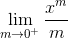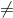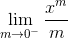limit DNE and give up.

But here's the idea why not find the average of the two limits.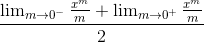we need to put the limits together, so we replacewith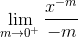Now that the limits are the same, we plug it back into the average equation: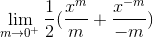=which is my original formula.

Last edited:
Well the obvious problem is that the formula
##\lim_{x\to a} \left( f(x)+g(x)\right) = \lim_{x\to a} f(x) + \lim_{x\to a} g(x)##
is only allowed if ##\lim_{x\to a} f(x)## and ##\lim_{x\to a} g(x)## individually exist, and in this case they don't.

Honestly I've seen less rigorous proofs in textbooks. but ok, suppose i "got the right answer the wrong way"
what is this formula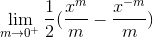=ln(x) called? how would it be "properly" derived?
added another graph for the skeptics. notice the size of the tick marks.

whats this Fourier series method called? I am interested

#### Attachments

what is this formula=ln(x) called?
I don't think it has a name.

how would it be "properly" derived?
No idea. My initial thought was L'Hopital, but I couldn't get it to work.

whats this Fourier series method called? I am interested

If ##f(x)## is a periodic function then under relatively weak conditions there exists constants ##a_n## and ##b_n## such that
##f(x) = \sum_{n=\infty}^\infty a_n \sin(2\pi nx/L) + b_n \cos(2\pi nx/L)##
where L is the period.
Importantly if ##f(x_0)## is a jump discontinuity then the Fourier series converges to ##\frac{f(x_0+) +f(x_0 -)}{2}## which is similar expression to what you have.

Hi DivergentSpectrum !with n tending to -1 (or m=n+1 tending to 0) is good in essence. But you should not use an indefinite integral.
The value of the integral is not xm/m , but is (xm-x0m)/m where x0 is the lower bound.
For m tending to 0 it tends to (1-1)/0 = 0/0 at first sight. But a more correct expression is :
(em ln(x) - em ln(x0))/m = (1+m ln(x)+O(m2)... -1-m ln(x0)-O(m2))/m = ln(x)-ln(x0)+O(m)
which limit is well defined = ln(x)-ln(x0)
The definition of the natural logarithm is stated with x0=1 so that ln(1)=0.
So, the definite integral tends to ln(x) as you claimed it.

•pwsnafu
you can also work backwards to find the derivative:
d/dx=
lim (xm-1+x-m-1)/2
m→0

=(x-1+x-1)/2
=x-1
qed.

therefore ln(x)==∫x-1 dx

I think if you were into numerically calculating ln(x), the limit definition would be immensely better than the taylor series.

I really doubt i discovered something new, but its still a really cool result.

edit: in my first proof i said
notice that if m was positive:
we would have a positive when x is positive; and a negative when x is negative

but if m was negative:
we would have a positive when x is negative; or a negative when x is positive.
So, you could say:
i think i meant to say if x is negative we would get some complex number, doesn't really affect anything though.

Last edited:
if you want a general rule just write

$$\int \! x^a\dfrac{\mathrm{d}x}{x}=C+\lim_{t\rightarrow a} \dfrac{x^t-1}{t}$$

I think if you were into numerically calculating ln(x), the limit definition would be immensely better than the taylor series.
How would you calculate ##x^{m}## faster than a Taylor series? A Taylor series only needs to worry about ##x^k## for integer k. You are asking for 1/m th roots.

Edit: this is really a moot question: calculators don't use the Taylor series of Log anyway.

im not sure entirely how floating point arithmetic works, but it converges everywhere as opposed to a series which is only good in the general area.
Regardless, its a lot "prettier" than a taylor series lol.
This definitely qualifies as a mathematical beauty type of thing. Personally it doesn't surprise me the method of averaging limits works for terms of sin and cos as well, considering how theyre so closely related to exp/ln. I guess you could almost say it "had to be true" that ln(x) is some kind of limiting case of the power rule. but im not going to go as far to say ln(x)=x0/0, lest i get stoned to death or burnt at the stake or something :P (maybe that's why i got a bad reception at first).

Maxima recognizes the limit as ln(x), so I am guessing mathematica does as well. What i don't get is why i can't find a single web page that states this formula

http://functions.wolfram.com/ElementaryFunctions/Log/09/

$$e^x=\lim_{h\rightarrow 0}(1+h\, x)^{1/h}\\ \log(x)=\lim_{h\rightarrow 0}\dfrac{x^h-1}{h}$$

Are dual limits. Both are well known. I will say a quick web search finds the first limit more often then the second. That is an interesting curiosity.

im not sure entirely how floating point arithmetic works, but it converges everywhere as opposed to a series which is only good in the general area.
This is not actually a problem because of ##\log(a 10^b) = \log(a) + b \log(10)##. Wikipedia has a nice explanation. See the "more efficient series" section.

Regardless, its a lot "prettier" than a taylor series lol. This definitely qualifies as a mathematical beauty type of thing.
Oh I know. Which I why I got out of computational mathematics :w

Personally it doesn't surprise me the method of averaging limits works for terms of sin and cos as well, considering how theyre so closely related to exp/ln.
I never thought of that, but Fourier series can be written in a more compact form as ##\sum_{n=0}^{\infty} c_n e^{inx}##, so yeah it makes sense.

I still see no proper proof.
An interesting curiosity, but I can't think of any practical uses for it. Or if there could even be any.

setting the fomula lurflurf gave, and my formula equal to each other: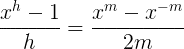i wonder what happens if you manage to solve for h. surely it would be the value of h required to match my approximation formula, and with that you could derive the error term. (assuming the error term of the left one is known)

An interesting curiosity, but I can't think of any practical uses for it. Or if there could even be any.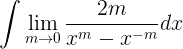lol

Last edited:
$$\dfrac{x^h-1}{h}=\dfrac{\exp(h\log(x))-1}{h}=\dfrac{1}{h}\left(\sum_{k=0}^\infty \dfrac{h^k\log^k(x)}{k!}-1\right)=\sum_{k=0}^\infty \dfrac{h^k\log^{k+1}(x)}{(k+1)!}$$

$$\dfrac{x^h-1}{h}=\dfrac{\exp(h\log(x))-1}{h}=\dfrac{1}{h}\left(\sum_{k=0}^\infty \dfrac{h^k\log^k(x)}{k!}-1\right)=\sum_{k=0}^\infty \dfrac{h^k\log^{k+1}(x)}{(k+1)!}$$
then as h goes to zero, only 0^0=1 remains, giving ln(x)
nice.
But are you sure this is the same limit as mine? they converge at different rates...

just realized the same idea will work: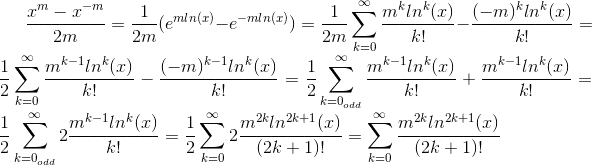then in the limit as m goes to zero, only 0^0=1 remains, giving just the first term, which is also ln(x)

Last edited:

Here i graphed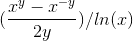the red line is pointing in the direction of x, the green line is pointing in the direction of y, and blue points in the direction of z.
Sorry if its hard to see.(i used png this time)

But there appears to be a "sweet spot" around x=1. makes sense considering that's ln(1)=0 and you don't even have to take a limit to get that.
But what makes my formula converge faster than the other one?

#### Attachments

$$\left(\dfrac{x^m-x^{-m}}{2m}\right)=x^{-m}\left(\dfrac{x^{2m}-1}{2m}\right)$$

$$\left(\dfrac{x^m-x^{-m}}{2m}\right)=x^{-m}\left(\dfrac{x^{2m}-1}{2m}\right)$$
hmm... this doesn't really explain convergence rates though

you can think of your limit as an average of
$$\dfrac{x^m-1}{m}\\ \text{and }\\ \dfrac{1-x^{-m}}{m}$$
one is and over estimate and one is an under estimate so the average is better than either
this is an example of estimating derivatives
where we have
$$\dfrac{\mathrm{f}(x+h)-\mathrm{f}(x)}{h}\\ \dfrac{\mathrm{f}(x)-\mathrm{f}(x-h)}{h}\\ \dfrac{\mathrm{f}(x+h)-\mathrm{f}(x-h)}{2h}$$

you can think of your limit as an average of
$$\dfrac{x^m-1}{m}\\ \text{and }\\ \dfrac{1-x^{-m}}{m}$$
one is and over estimate and one is an under estimate so the average is better than either
this is an example of estimating derivatives
where we have
$$\dfrac{\mathrm{f}(x+h)-\mathrm{f}(x)}{h}\\ \dfrac{\mathrm{f}(x)-\mathrm{f}(x-h)}{h}\\ \dfrac{\mathrm{f}(x+h)-\mathrm{f}(x-h)}{2h}$$
ahh that makes sense. Funny how there can be so many interpretations of one thing
So I am guessing there must be a way to generalize limit formulas to an arbitrary order?

Last edited:
I always found the relationship ## \int_1^x \frac{1}{x'}dx'=\ln x ## amazing. Think about it: The area under the function of inverse numbers from 1 to x tells you what exponent you have to use for e to get x. I also always wondered if there is a way to understand this more intuitively.

this is an example of estimating derivatives
derivative of what?
Is it possible to take a limit and increase its convergence to arbitrary order n? like with numerical differentation/integration?

edit: applied it to the e^x limit:

if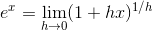then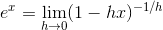i checked one is an overestimation and the other is an underestimation
so a better estimate would be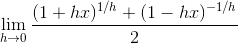unfortunately its no where near as elegant as the expression for ln because the radicals can't be combined.
Its funny, i always felt like ln was a "boring" function for some reason. But lately I've got a newfound respect for it.

Last edited:
$$\log(x)=\left. \dfrac{d}{dm}x^m\right|_{m=0}=\lim_{h\rightarrow 0}\dfrac{x^{0+h}-x^0}{h}=\lim_{h\rightarrow 0}\dfrac{x^h-1}{h}$$

yes you can increase the order as much as you want. The trouble is unless you do some clever stuff high order approximate differentiation is unstable.